Courses

# Heat Pump & Refrigeration Cycles & Systems - MCQ Test 2

## 30 Questions MCQ Test RRB JE for Mechanical Engineering | Heat Pump & Refrigeration Cycles & Systems - MCQ Test 2

Description
This mock test of Heat Pump & Refrigeration Cycles & Systems - MCQ Test 2 for Mechanical Engineering helps you for every Mechanical Engineering entrance exam. This contains 30 Multiple Choice Questions for Mechanical Engineering Heat Pump & Refrigeration Cycles & Systems - MCQ Test 2 (mcq) to study with solutions a complete question bank. The solved questions answers in this Heat Pump & Refrigeration Cycles & Systems - MCQ Test 2 quiz give you a good mix of easy questions and tough questions. Mechanical Engineering students definitely take this Heat Pump & Refrigeration Cycles & Systems - MCQ Test 2 exercise for a better result in the exam. You can find other Heat Pump & Refrigeration Cycles & Systems - MCQ Test 2 extra questions, long questions & short questions for Mechanical Engineering on EduRev as well by searching above.
QUESTION: 1

### A heat pump working on a reversed Carnot cycle has a C.O.P. of 5. lf it works as a refrigerator taking 1 kW of work input, the refrigerating effect will be:

Solution: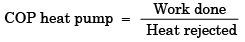or heat rejected = 5 × work done

And heat rejected = refrigeration effect + work input

or, 5 × work input – work input = refrigeration effect

or, 4 × work input = refrigeration effect

or refrigeration effect = 4 × 1 kW = 4 kW

QUESTION: 2

### If an engine of 40 percent thermal efficiency drives a refrigerator having a coefficient of performance of 5, then the heat input to the engine for each kJ of heat removed from the cold body of the refrigerator is:

Solution: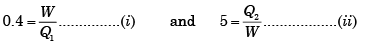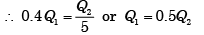QUESTION: 3

### A refrigerator works on reversed Carnot cycle producing a temperature of – 40°C. Work done per TR is 700 kJ per ten minutes. What is the value of its COP?

Solution: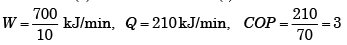QUESTION: 4

In a refrigeration plant, if the condenser temperature increases, the power input to the compressor will

Solution:
QUESTION: 5

The COP of a Carnot heat pump used for heating a room at 20°C by exchanging heat with river water at 10°C is:

Solution: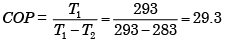QUESTION: 6

A refrigerating machine working on reversed Carnot cycle consumes 6kW to produce a refrigerating effect of 1000kJ/min for maintaining a region at – 40oC.The higher temperature (in degree centigrade) of the cycle will be:

Solution: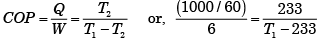or, T − 233= 83.88 or,T = 316.88 K = 43.88°C

QUESTION: 7

A Carnot refrigerator works between the temperatures of 200 K and 300 K. If the refrigerator receives 1 kW of heat the work requirement will be:

Solution: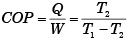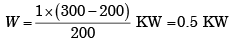QUESTION: 8

Operating temperature of a cold storage is –2°C From the surrounding at ambient temperature of 40°C heat leaked into the cold storage is 30 kW. If the actual COP of the plant is 1/10th of the maximum possible COP, then what will be the power required to pump out the heat to maintain the cold storage temperature at –2°C?

Solution: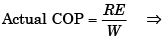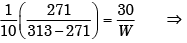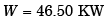QUESTION: 9

One ton refrigeration is equivalent to:

Solution:
QUESTION: 10

When the lower temperature is fixed, COP of a refrigerating machine can be improved by:

Solution:

In heat engines higher efficiency can be achieved when (T1 – T2) is higher. In refrigerating machines it is the reverse, i.e. (T1 – T2) should be lower.

QUESTION: 11

A refrigerating machine working on reversed Carnot cycle takes out 2 kW of heat from the system at 200 K while working between temperature limits of 300 K and 200 K. COP and power consumed by the cycle will, respectively, be:

Solution: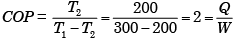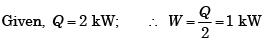QUESTION: 12

In the system given above, the temperature T = 300 K. When is the thermodynamic efficiency σE of engine E equal to the reciprocal of the COP of R?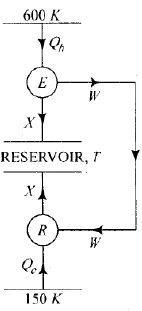Solution: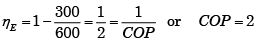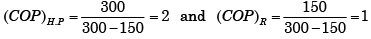∴ Rmust act as a Heat pump

QUESTION: 13

The COP of a Carnot heat pump used for heating a room at 20°C by exchanging heat with river water at 10°C is:

Solution: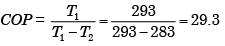QUESTION: 14

A refrigeration system operates on the reversed Carnot cycle. The temperature for the system is: Higher temperature = 40°C and Lower temperature = 20°C.

The capacity of the refrigeration system is 10 TR. What is the heat rejected from the system per hour if all the losses are neglected?

Solution: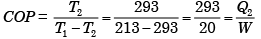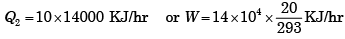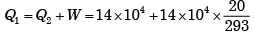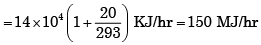QUESTION: 15

Assertion (A ): T he COP of an air-conditioning plant is lower than that of an ice plant.

Reason (R): The  tem peratures required in the ice plant are lower than those required for an air-conditioning plant.

Solution:

The COP of an air-conditioning plant is higher than that of an ice plant.

QUESTION: 16

For a given value of TH (Source temperature) for a reversed Carnot cycle, the variation of TL (Sink temperature) for different values of COP is represented by which one of the following graphs?

Solution: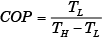COP is on y-axis and TL on x-axis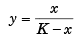⇒ Curve (C) is the corerct representation of above equations since it passes through the origin

QUESTION: 17

Efficiency of a Carnot engine is 75%. If the cycle direction is reversed, COP of the reversed Carnot cycle is

Solution: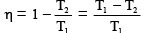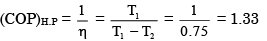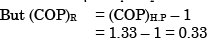If the cycle direction is reversed it will be a heat pump not refrigerator. Students make a common mistake here and calculated (COP)R. We know that the definition of refrigeration is producing a temperature below atmospheric temperature. In heat engine lower temperature is atmospheric temperature. When we reverse this cycle then lower temperature will be atmospheric and higher temperature will be more than atmospheric means it will be a Heat Pump not a refrigerator.

QUESTION: 18

A Carnot heat pump works between temperature limits of 277º C and 27º C. Its COP is

Solution: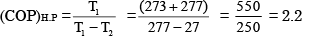[We may put T1 and T2 in ºC or in K but T1 – T2 will be same]

QUESTION: 19

A refrigerator works on reversed Carnot cycle producing a temperature of – 40°C. Work done per TR is 700 kJ per ten minutes. What is the value of its COP?

Solution: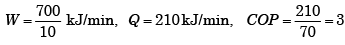QUESTION: 20

In the above figure, E is a heat engine with efficiency of 0.4 and R is a refrigerator. Given that Q2 + Q4 = 3Q1 the COP of the refrigerator is: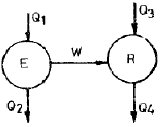Solution:

For heat engine, efficiency =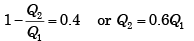And for refrigerator,

W + Q = Q4    or (Q1 – Q2) + Q3 = Q4 or Q1  + Q3 = Q2 + Q4 = 3Q1

Therefore  2 Q1 = Q3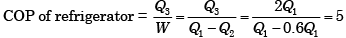QUESTION: 21

The coefficient of performance (COP) of a refrigerator working as a heat pump is given by:

Solution:

The COP of refrigerator is one less than COP of heat pump, if same refrigerator starts working as heat pump i.e. (COP)heat pump = (COP)refrigerator + 1

QUESTION: 22

A refrigerator operates between the temperatures of –23ºC and 27ºC. If one TR = 3.5 kW, the minimum power required per TR to operate the refrigerator is

Solution: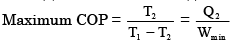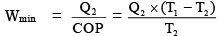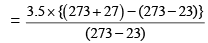= 0.7 kW

QUESTION: 23

Which one of the following statements is correct?

In a domestic refrigerator periodic defrosting is required because frosting

Solution:
QUESTION: 24

Consider the following statements:  In the thermoelectric refrigeration, the coefficient of performance is a function of:

1. Electrical conductivity of materials

2. Pelti er coefficie nt

3. Seeb eck coefficie nt

4. Temperature at cold and hot junctions

5. Thermal conductivity of materials.

Of these statements:

[IES-1997]

Solution:
QUESTION: 25

In a reversible cycle, the source temperature is 227°C and the sink temperature is 27°C. The maximum available work for a heat input of 100 kJ will be:

Solution:

Maximum efficiency for 227° and 27°C sources =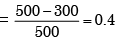Maximum work available for a heat input of 100 kJ  = 0.4 × 100 = 40 kJ.

QUESTION: 26

In a 0.5 TR capacity water cooler, water enters at 30°C and leaves at 15°C.What is the actual water flow rate?

Solution: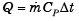or 0.5 12660 4.2 (30 15 ) or 100 kg/hr

QUESTION: 27

It is proposed to build refrigeration plant for a cold storage to be maintained at – 3°C. The ambient temperature is 27°C. If 5 × 106 kJ/h of energy is to be continuously removed from the cold storage, the MINIMUM power required to run the refrigerator will be:

Solution: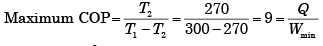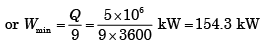QUESTION: 28

Assertion (A ): When solid CO2 (dry ice) is exposed to the atmosphere, it gets transformed directly into vapour absorbing the latent heat of sublimation from the surroundings

Reason (R): The triple  point of CO2 is at about 5 atmospheric pressure and at 216 K.

Solution:
QUESTION: 29

In a one ton capacity water cooler, water enters at 30°C at the rate of 200 litres per hour. The outlet temperature of water will be (sp. heat of water = 4.18 kJ/kg K)

Solution:

3.516 × 36 00 = 4.18× 200 × (300 − x )

or x   = 14 .98°C ≈ 15 °C

QUESTION: 30

A heat pump is shown schematically as

Solution:

In heat pump, heat is rejected to source, work done on compressor, and heat absorbed from sink.